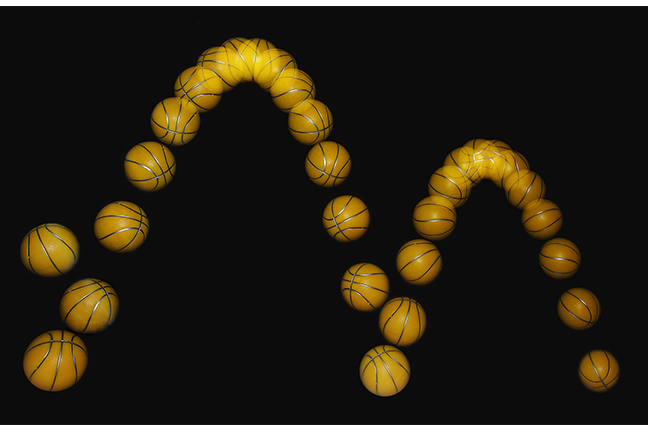## Want to keep learning?

This content is taken from the UNSW Sydney's online course, Maths for Humans: Linear, Quadratic & Inverse Relations. Join the course to learn more.
2.21

## UNSW SydneyHow high the ball?

# Vertices, axes and maximum values

How high does a basketball go? Because of the ubiquity of quadratic curves in motion and other applications, it is useful to be able to determine its maximum or minimum value. Al Khwarizmi’s formula is the algebraic key that unlocks the symmetry and position of a general parabola through understanding its vertex and axis.

In this step we will

• see how Khwarizmi’s formula determines the vertex and axis of a parabola

• show how to accurately predict the maximum height of a quadratic function.

## The vertex and axis of a parabola

Recall that by translating a standard parabola $$\normalsize{y = ax^2}$$ to the form $$\normalsize{y = a(x-h)^2+k}$$ we determined that its vertex was the point $$\normalsize{[h,k]}$$.

We are now going to see how to find the vertex of a general parabola $${\normalsize p(x) =ax^2+bx+c }$$. Al Khwarizmi’s formula

$\Large{ax^2+bx+c=a\left(x+\frac{b}{2a}\right)^2+\frac{4ac-b^2}{4a}}. \label{1} \tag{1}$

is a rewrite of the general quadratic exactly in the form $$\normalsize{y = a(x-h)^2+k}$$, which immediately allows us to see that the vertex of the associated parabola is the point

$\Large{[h,k] = \Bigg[-\frac{b}{2a},\frac{4ac-b^2}{4a}\Bigg]} .$

We also deduce that the axis of symmetry of the parabola, which is the vertical line through the vertex, is

$\Large {x=-\frac{b}{2a}}.$

Since the square term $${\normalsize \left(x+\frac{b}{2a}\right)^2 \geq 0 }$$, from $$\normalsize{(\ref{1})}$$ we deduce that if $$a>0$$ then the minimum value of $$p(x)$$ is

$\Large {\frac{4ac-b^2}{4a}}.$

Similarly if $$a< 0$$ then the maximum possible value of $$p(x)$$ is the same quantity $$\normalsize{\frac{4ac-b^2}{4a}}.$$

Q1 (E): Where is the vertex and axis of the parabola $$\normalsize{y=-4(x-3)^2+5}$$ ?

Q2 (E): Find the vertex and axis of $$\normalsize{y=3x^2+4x+7 }$$.

Q3 (M): What is the maximum value of the polynomial function $$\normalsize{p(x)=-2x^2+3x-5 }$$. What is the minimum value?

Q4 (C): Suppose that $$\normalsize a<0$$. Show that if $$\normalsize d > c - \frac{b^2}{4a}$$ then there are no solutions to the equation $${\normalsize ax^2 + bx + c = d }.$$

A1. The parabola $$\normalsize{y=-4(x-3)^2+5}$$ has vertex $$\normalsize{[3,5]}$$ and axis the line $$\normalsize{x=3}$$.

A2. Let’s find al-Khwarizmi’s identity for $$\normalsize p(x)=3x^2+4x+7$$. We complete the square as follows: (please study this carefully!)

\Large{\begin{align} p(x)&=3\left(x^2+\frac{4}{3}x+\frac{7}{3}\right)\\ &= 3\left(x^2+\frac{4}{3}x+\Bigg(\frac{2}{3}\Bigg)^2+\frac{7}{3}-\Bigg(\frac{2}{3}\Bigg)^2\right)\\ &=3\bigg(\bigg(x+\frac{2}{3}\bigg)^2+\frac{17}{9}\bigg) \\ &=3\Bigg(x+\frac{2}{3}\Bigg)^2+\frac{17}{3}. \end{align}}

The vertex of the parabola is then the point $$\normalsize \big[-\frac{2}{3},\frac{17}{3}\big]$$. The axis is the line

$$\Large x=-\frac{2}{3}$$.A3. For the polynomial $$\normalsize{p(x)=-2x^2+3x-5 }$$, the coefficient of $${\normalsize x^2}$$ is negative. So it has a maximum value which is

$\Large {\frac{4ac-b^2}{4a}=\frac{4(-2)(-5)-3^2}{4(-2)}=-\frac{31}{8}.}$

As for a minimum value, there is none, as the branches of the parabola become increasingly more negative for large values of $$\normalsize x$$.A4. Suppose $$\normalsize ax^2 + bx + c = d > c - \frac{b^2}{4a}$$ where $$\normalsize a<0$$. This can be rearranged as

${\Large x^2 + \frac{b}{a}x + \left(\frac{b}{2a}\right)^2 < 0}.$

But the left hand side is a perfect square

${\Large \left(x + \frac{b}{2a}\right)^2 < 0 }$

and square numbers cannot be negative. Hence there can be no solutions to $$\normalsize ax^2 + bx + c = d$$ when $$\normalsize d > c - \frac{b^2}{4a}$$.